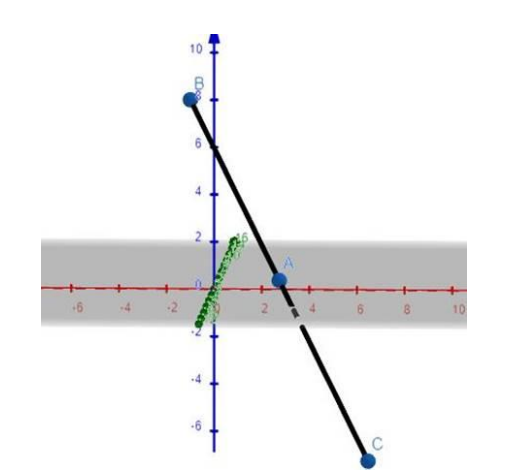# Show that the following points are collinear :

Question:

Show that the following points are collinear :

$A(3,-5,1), B(-1,0,8)$ and $C(7,-10,-6)$

Solution:

To prove: the 3 points are collinear.

Formula: The distance between two points $\left(\mathrm{x}_{1}, \mathrm{y}_{1}, \mathrm{Z}_{1}\right)$ and $\left(\mathrm{x}_{2}, \mathrm{y}_{2}, \mathrm{Z}_{2}\right)$ is given by

$\mathrm{D}=\sqrt{\left(x_{2}-x_{1}\right)^{2}+\left(y_{2}-y_{1}\right)^{2}+\left(z_{2}-z_{1}\right)^{2}}$

Here,

$\left(x_{1}, y_{1}, z_{1}\right)=(3,-5,1)$

$\left(x_{2}, y_{2}, z_{2}\right)=(-1,0,8)$

$\left(x_{3}, y_{3}, z_{3}\right)=(7,-10,-6)$

Length $\mathrm{AB}=\sqrt{\left(x_{2}-x_{1}\right)^{2}+\left(y_{2}-y_{1}\right)^{2}+\left(z_{2}-z_{1}\right)^{2}}$

$=\sqrt{(-1-3)^{2}+(0+5)^{2}+(8-1)^{2}}$

$=\sqrt{(-4)^{2}+(5)^{2}+(7)^{2}}$

$=\sqrt{16+25+49}$

$=\sqrt{90}=3 \sqrt{10}$

Length $\mathrm{BC}=\sqrt{\left(x_{3}-x_{2}\right)^{2}+\left(y_{3}-y_{2}\right)^{2}+\left(z_{3}-z_{2}\right)^{2}}$

$=\sqrt{(7+1)^{2}+(-10-0)^{2}+(-6-8)^{2}}$

$=\sqrt{(8)^{2}+(-10)^{2}+(-14)^{2}}$

$=\sqrt{64+100+196}$

$=\sqrt{360}=6 \sqrt{10}$

Length $\mathrm{AC}=\sqrt{\left(x_{3}-x_{1}\right)^{2}+\left(y_{3}-y_{1}\right)^{2}+\left(z_{3}-z_{1}\right)^{2}}$

$=\sqrt{(7-3)^{2}+(-10+5)^{2}+(-6-1)^{2}}$

$=\sqrt{(4)^{2}+(-5)^{2}+(-7)^{2}}$

$=\sqrt{16+25+49}$

$=\sqrt{90}=3 \sqrt{10}$

$B A+B C=3 \sqrt{10}+3 \sqrt{10}=6 \sqrt{10}=B C$

Therefore $A, B, C$ are collinear.